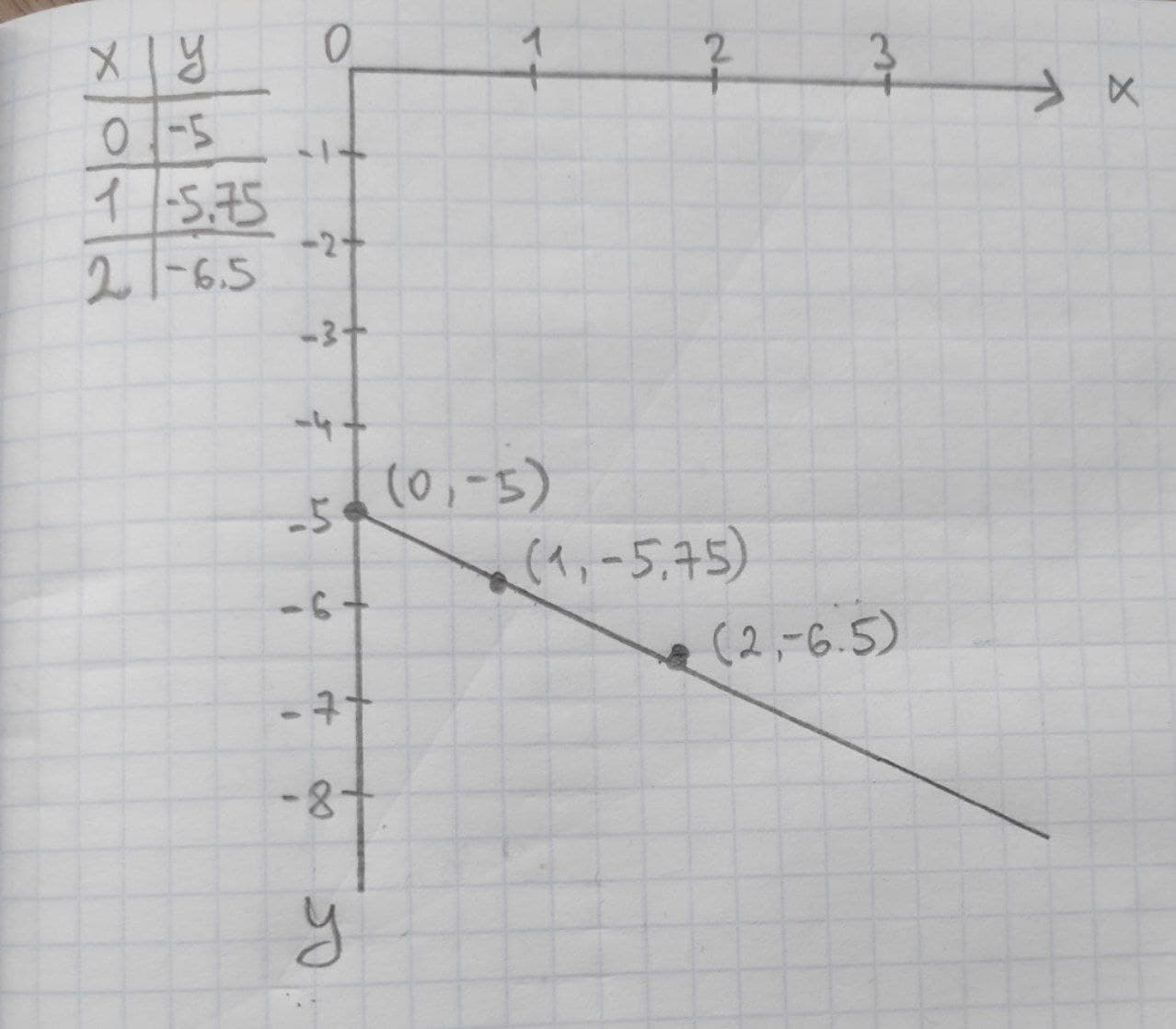Braxton Pugh

2020-11-05

Given linear equation y = -0.75x-5
a. find the y-intercept and slope.
b. determine whether the line slopes upward, slopes downward, or is horizontal, without graphing the equation.
c. use two points to graph the equation.unett

Expert

(a) $y={b}_{0}+{b}_{1}x$
where ${b}_{0}$ is the y intercept
${b}_{1}$ is the slope
Slope = -0.75
y-intercept = -5
from the linear equation
${b}_{0}=-5$
${b}_{1}=-0.75$
(b) The slope is downward in graph. Because, the slope is negative value.
(c) Two point to graph:Do you have a similar question?